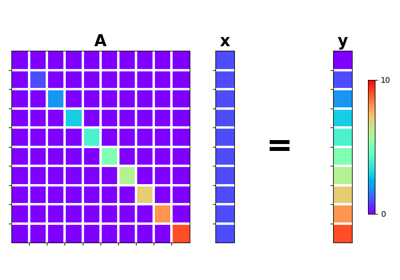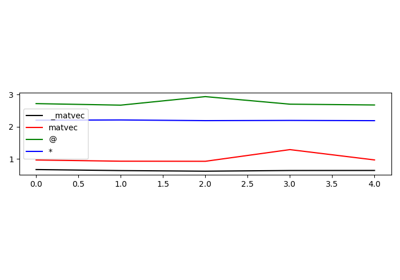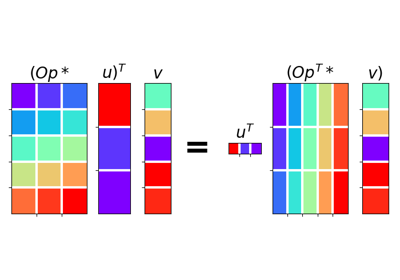# pylops.Diagonal#

class pylops.Diagonal(diag, dims=None, axis=-1, dtype='float64', name='D')[source]#

Diagonal operator.

Applies element-wise multiplication of the input vector with the vector diag in forward and with its complex conjugate in adjoint mode.

This operator can also broadcast; in this case the input vector is reshaped into its dimensions dims and the element-wise multiplication with diag is perfomed along axis. Note that the vector diag will need to have size equal to dims[axis].

Parameters
diagnumpy.ndarray

Vector to be used for element-wise multiplication.

dimslist, optional

Number of samples for each dimension (None if only one dimension is available)

axisint, optional

New in version 2.0.0.

Axis along which multiplication is applied.

dtypestr, optional

Type of elements in input array.

namestr, optional

New in version 2.0.0.

Name of operator (to be used by pylops.utils.describe.describe)

Notes

Element-wise multiplication between the model $$\mathbf{x}$$ and/or data $$\mathbf{y}$$ vectors and the array $$\mathbf{d}$$ can be expressed as

$y_i = d_i x_i \quad \forall i=1,2,\ldots,N$

This is equivalent to a matrix-vector multiplication with a matrix containing the vector $$\mathbf{d}$$ along its main diagonal.

For real-valued diag, the Diagonal operator is self-adjoint as the adjoint of a diagonal matrix is the diagonal matrix itself. For complex-valued diag, the adjoint is equivalent to the element-wise multiplication with the complex conjugate elements of diag.

Attributes
shapetuple

Operator shape

explicitbool

Operator contains a matrix that can be solved explicitly (True) or not (False)

Methods

 __init__(diag[, dims, axis, dtype, name]) adjoint() apply_columns(cols) Apply subset of columns of operator cond([uselobpcg]) Condition number of linear operator. conj() Complex conjugate operator div(y[, niter, densesolver]) Solve the linear problem $$\mathbf{y}=\mathbf{A}\mathbf{x}$$. dot(x) Matrix-matrix or matrix-vector multiplication. eigs([neigs, symmetric, niter, uselobpcg]) Most significant eigenvalues of linear operator. matmat(X) Matrix-matrix multiplication. matrix() Return diagonal matrix as dense numpy.ndarray matvec(x) Matrix-vector multiplication. reset_count() Reset counters rmatmat(X) Matrix-matrix multiplication. rmatvec(x) Adjoint matrix-vector multiplication. todense() Fast implementation of todense based on known structure of the operator toimag([forw, adj]) Imag operator toreal([forw, adj]) Real operator tosparse() Return sparse matrix. trace([neval, method, backend]) Trace of linear operator. transpose()

## Examples using pylops.Diagonal#Describe

DescribeDiagonal

Diagonal01. The LinearOperator

01. The LinearOperator02. The Dot-Test

02. The Dot-Test